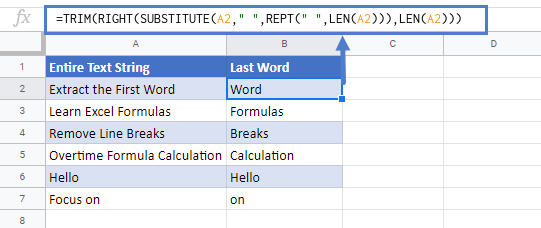# Extract the Last Word In Excel & Google Sheets

This tutorial will demonstrate how to extract the last word from a cell in Excel & Google Sheets.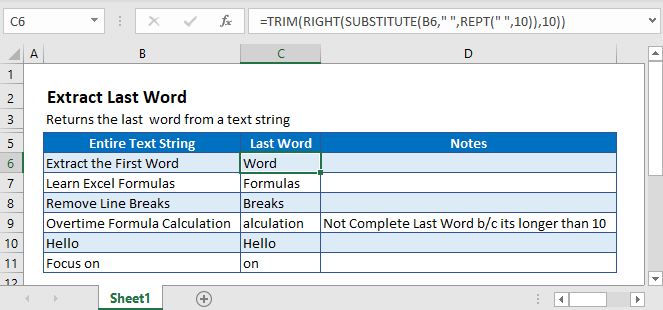## Extract Last Word

If we wanted to extract the first word of a cell, we could simply use the FIND Function to find the first space and the LEFT Function to output the word before the space.

Unfortunately, Excel doesn’t have a reverse FIND Function, so instead we will need to develop a different solution:

1. Use the SUBSTITUTE Function to replace the spaces in between words with a large number (n) of spaces.
2. Use the RIGHT Function to calculate the right n number of spaces. (This will include our word, as well as a number of additional spaces)
3. Use the TRIM Function to trim out the extra spaces, leaving only the last word.

Here is the formula we will use:

``=TRIM(RIGHT(SUBSTITUTE(B3," ",REPT(" ",10)),10))``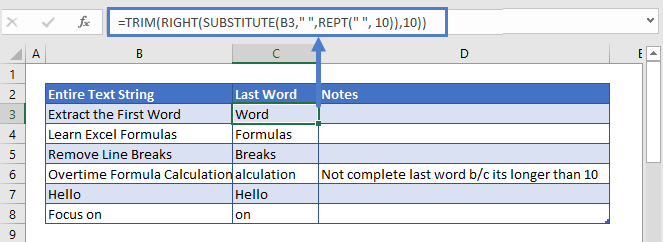Notice for this example we choose n=10. This number is too small: Cell C6 doesn’t contain the full last word because the word is 11 characters long.

We’ve chosen a small number for demonstration purposes, but we recommend that you use a much larger number (ex. 99) or use the versatile formula found at the end of this tutorial.

Now let’s walk through the formula:

### REPT Function

The REPT Function repeats a character (n) number of times. This formula will generate 10 spaces:

``=REPT(" ",10)``

Here to show who this function will work, we have replaced the blank spaces with dashes (-):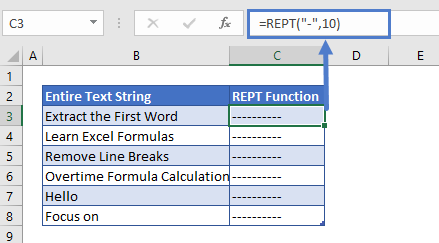### SUBSTITUTE Function

The SUBSTITUTE function finds all the blank spaces in the text string and replaces the blank spaces with 10 spaces.

``=SUBSTITUTE(B3," ",C3)``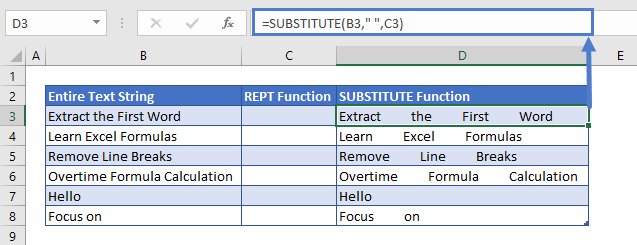### RIGHT Function

The RIGHT function extracts the last 10 characters from the cell:

``=RIGHT(D3,10)``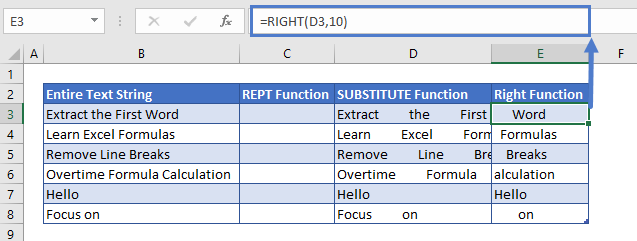### TRIM Function

The TRIM function removes all the leading and trailing spaces from the text and returns only the last word:

``=TRIM(E3)``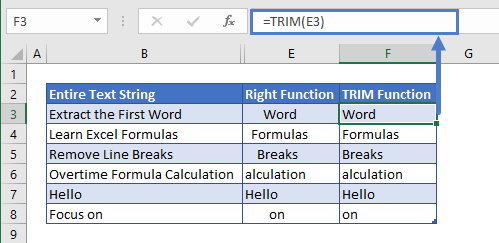## Versatile Formula

Instead of defining the number (n), you can use the LEN Function to calculate n as the number of characters in the cell. This formula will work regardless of how big the last word is.

``=TRIM(RIGHT(SUBSTITUTE(B3," ",REPT(" ",LEN(B3))),LEN(B3)))``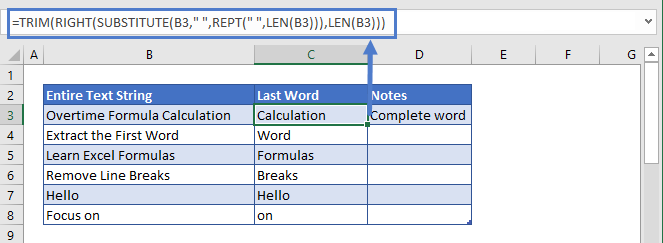### Easy Excel Automation